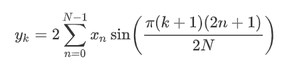Open in App
Not now

# Python – scipy.fft.dst() method

• Last Updated : 01 Oct, 2020

With the help of scipy.fft.dst() method, we can compute the discrete sine transform by selecting different types of sequences and return the transformed array by using this method.Syntax :

```scipy.fft.dst(x, type=2)
```

Return value: It will return the transformed array.

Example #1: In this example, we can see that by using scipy.fft.dst() method, we are able to get the discrete sine transform by selecting different types of sequences by default it’s 2.

## Python3

 `# import scipy ` `from` `scipy ``import` `fft ` ` `  `# Using scipy.fft.dst() method ` `gfg ``=` `fft.dst([``1``, ``2``, ``3``, ``4``]) ` ` `  `print``(gfg)`

Output :

```[13.06562965 -5.65685425  5.41196100 -4.00000000]
```

Example #2 :

## Python3

 `# import scipy ` `from` `scipy ``import` `fft ` ` `  `# Using scipy.fft.dst() method ` `gfg ``=` `fft.dst([``-``6``, ``5``, ``-``4``, ``3``, ``-``2``, ``1``], ``3``) ` ` `  `print``(gfg)`

Output :

```[ -1.43023367  -2.3137085   -6.16568427  -7.77337942 -20.3137085
-23.82253852]
```
My Personal Notes arrow_drop_up
Related Articles